JEE  >  NCERT Exemplar (Part - 2) - Solutions

# NCERT Exemplar (Part - 2) - Solutions Notes | Study Additional Documents and Tests for JEE - JEE

## Document Description: NCERT Exemplar (Part - 2) - Solutions for JEE 2022 is part of Additional Documents and Tests for JEE preparation. The notes and questions for NCERT Exemplar (Part - 2) - Solutions have been prepared according to the JEE exam syllabus. Information about NCERT Exemplar (Part - 2) - Solutions covers topics like and NCERT Exemplar (Part - 2) - Solutions Example, for JEE 2022 Exam. Find important definitions, questions, notes, meanings, examples, exercises and tests below for NCERT Exemplar (Part - 2) - Solutions.

Introduction of NCERT Exemplar (Part - 2) - Solutions in English is available as part of our Additional Documents and Tests for JEE for JEE & NCERT Exemplar (Part - 2) - Solutions in Hindi for Additional Documents and Tests for JEE course. Download more important topics related with notes, lectures and mock test series for JEE Exam by signing up for free. JEE: NCERT Exemplar (Part - 2) - Solutions Notes | Study Additional Documents and Tests for JEE - JEE
 1 Crore+ students have signed up on EduRev. Have you?

SHORT ANSWER TYPE QUESTIONS

Q.36. Components of a binary mixture of two liquids A and B were being separated by distillation. After some time separation of components stopped and composition of vapour phase became same as that of liquid phase. Both the components started coming in the distillate. Explain why this happened.
Ans. Both the components are appearing in the distillate and composition of liquid and vapour is same. This shows that liquids have formed azeotropic mixture and boil at constant temperature hence cannot be separated at this stage by distillation or fractional distillation. Solution having azeotropic nature shows large positive or negative deviation from Raoult's law depending upon intermolecular interaction.

Q.37. Explain why on addition of 1 mol of NaCl to 1 litre of water, the boiling point of water increases, while addition of 1 mol of methyl alcohol to one litre of water decreases its boiling point.
Ans. NaCI is a non-volatile solute. So, its addition to water lowers the vapour pressure of the water. Hence, boiling point of water (solution) increases. On the other hand, methyl alcohol is more volatile than water.
So, its addition to water increases the total vapour pressure over the solution. It results in the decrease of boiling point.

Q.38. Explain the solubility rule “like dissolves like” in terms of intermolecular forces that exist in solutions.
Ans. If the intermolecular interactions are similar in both constituents, i.e., solute and solvent then solute dissolves in the solvent.
Example: polar solutes dissolve in polar solvents and non-polar solutes in non-polar solvents.
Thus, the statement 'like dissolves like' proves to be true.
Example: organic compounds dissolve in non-polar organic solvent while polar inorganic compounds (salts) dissolve in polar solvent (water).

Q.39. Concentration terms such as mass percentage, ppm, mole fraction and molality are independent of temperature, however molarity is a function of temperature. Explain.
Ans. Molarity is defined as the number of moles of solute dissolved per litre of a solution. Since, volume depends on temperature and changes with change in temperature, the molarity will also change with change in temperature.
On the other hand, mass does not change with change in temperature, so other concentration terms given in the question also do not do so. Thus, temperature has no effect on the mass but it has significant effect on volume.

Q.40. What is the significance of Henry’s Law constant KH?
Ans. According to Henry's law
p ∝ x ⇒ p = KHx
Higher the value of Henry's law constant KH, the lower is the solubility of the gas in a liquid. Thus, the solubility of a gas in the given liquid can be increased by increasing pressure.

Q.41. Why are aquatic species more comfortable in cold water in comparison to warm water?
Ans. Aquatic species are more comfortable in cold water due to the presence of more oxygen. Solubility of oxygen in water increases with decrease in temperature as solubility of a gas in given liquid decreases with increase in temperature.

Q.42.
(a) Explain the following phenomena with the help of Henry’s law.
(i) Painful condition known as bends.
(ii) Feeling of weakness and discomfort in breathing at high altitude.
(b) Why soda water bottle kept at room temperature fizzes on opening?
Ans.
(a)
(i) Henry's law represents a relation between solubility of gases in liquid and pressure. Scuba drivers when come towards surface, the pressure gradually decreases. This reduced pressure releases the dissolved gas present in blood and leads to formation of bubbles of nitrogen in the blood. This creates a painful condition by blocking capillaries known as blends.
(ii) At high altitude atmospheric pressure is low as compared to surface which causes difficulty in breathing. In that condition we feel weakness and discomfort.
(b) Soda water bottle kept at room temperature fizzes on opening due to different pressure inside and outside the bottle. When the bottle is opened to air, the partial pressure of CO2 is above the solution decreases. As a result, solubility decreases and hence CO2 bubbles out with a fizz.

Q.43. Why is the vapour pressure of an aqueous solution of glucose lower than that of water?
Ans. In pure liquid, the entire surface of liquid is occupied by the molecules of water. When a non-volatile solute,
Example: glucose is dissolved in water.
the fraction of surface covered by the solvent molecules gets reduced because some positions are occupied by glucose molecules.
So, number of solvent molecules escaping from the surface is reduced. That is why vapour pressure of aqueous solution of glucose is reduced.

Q.44. How does sprinkling of salt help in clearing the snow covered roads in hilly areas? Explain the phenomenon involved in the process.
Ans. When salt is spread over snow covered roads, snow starts melting from the surface due to the depression in freezing point of water on addition of a non-volatile solute (salt) to it. It helps in clearing of roads.
Hence, the phenomenon involved is ‘depression in freezing point of water when a non volatile solute is dissolves in it.’

Q.45. What is “semi permeable membrane”?
Ans. Continuous sheets or films (natural or synthetic) which contain a network of submicroscopic holes or pores through which small solvent molecules like water can pass; but the passage of bigger molecules of solute is hindered, are known as semi permeable membrane.
Example: cellophane membrane.

Q.46. Give an example of a material used for making semipermeable membrane for carrying out reverse osmosis.
Ans. Since pressure required for the reverse osmosis is very high, so, a suitable material is used for making semipermeable membrane. It is generally cellulose acetate placed over suitable support.

MATCHING TYPE

Note : In the following questions match the items given in Column I and Column II.
Q.47. Match the items given in Column I and Column II.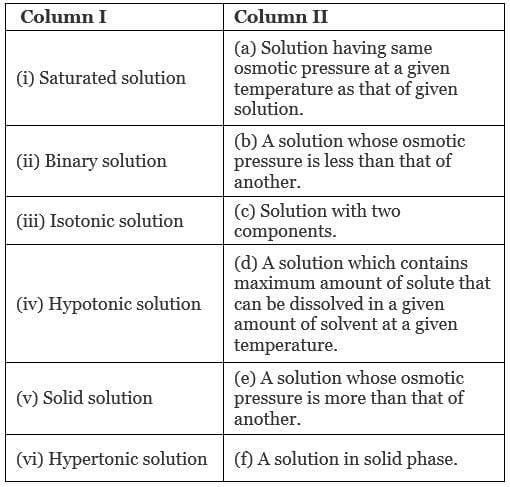Ans. (i → d), (ii → c); (iii → a); (iv → b), (v → f); (vi → e)
Solution.
(i) Saturated solution: A solution which contains maximum amounts of solute that can be dissolved in a given amount of solvent at a given temperature.
(ii) Binary solution: A solution with two components is known as binary solution.
(iii) Isotonic solution: A solution having same osmotic pressure at a given temperature as that of given solution is known as isotonic solution.
(iv) Hypotonic solution: A solution whose osmotic pressure is less than another is known as hypotonic solution.
(v) Solid solution: A solution in solid phase is known as solid solution.
(vi) Hypertonic solution: A solution whose osmotic pressure is greater than that of another is known as hypertonic solution.

Q.48. Match the items given in Column I with the type of solutions given in Column II.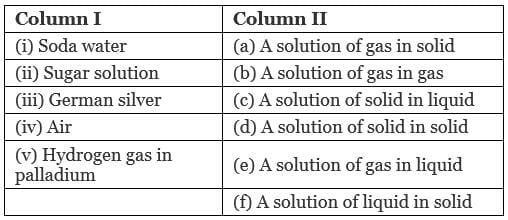Ans. (i → e), (ii → c), (iii → d); (iv → b), (v → a)
Solution.
(i) Soda water: A solution of gas in liquid.
Example: CO2 in soft drinks.
(ii) Sugar solution: A solution of solid in liquid in which sugar particles (soild) are dissolved in water (liquid).
(iii) German silver: German silver is an alloy which is a solid solution of solid in solid. It is an alloy of Cu, Zn and Ni.
(iv) Air: A solution of gas in gas. Air is a mixture of various gases.
(v) Hydrogen gas in palladium: This solution is an example of solution of gas in solid. This is used as an reducing agent.

Q.49. Match the laws given in Column I with expressions given in Column II.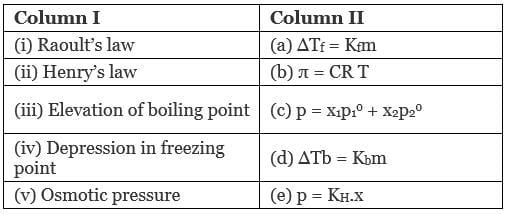Ans.  (i → c), (ii → e), (iii → a); (iv → d), (v → b)
Solution.
(a) Raoult's law: Mathematical representation of Raoult's law p = x1p10 + x2p02
(b) Henry's law: p = KH.x
(c) Elevation of boiling point: Mathematical representation, ∆Tb = Kbm
(d) Depression in freezing point: Mathematical representation, ∆Tf = Kfm
(e) Osmotic pressure: Mathematical representation, π = CRT

Q.50. Match the terms given in Column I with expressions given in Column II.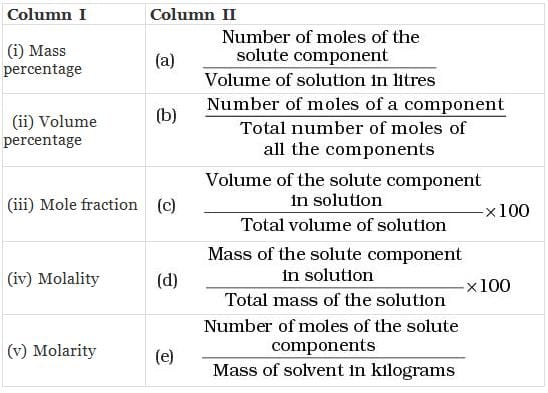Ans. (i → d), (ii →  c), (iii →  b), (iv →  e), (v → a)
Solution.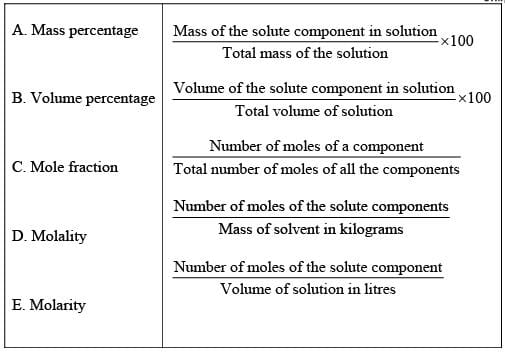ASSERTION AND REASON TYPE

Note : In the following questions a statement of assertion followed by a statement of reason is given. Choose the correct answer out of the following choices.
Q.51. Assertion: Molarity of a solution in liquid state changes with temperature.
Reason: The volume of a solution changes with change in temperature.
(i) Assertion and reason both are correct statements and reason is correct explanation for assertion.
(ii) Assertion and reason both are correct statements but reason is not correct explanation for assertion.
(iii) Assertion is correct statement but reason is wrong statement.
(iv) Assertion and reason both are incorrect statements.
(v) Assertion is wrong statement but reason is correct statement.

Ans. (i)
Solution.

Volume of solutions is a function of temperature which varies with temperature. Hence, molarity of solution in liquid state changes with temperature.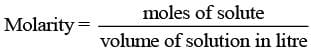Q.52. Assertion : When methyl alcohol is added to water, boiling point of water increases.
Reason : When a volatile solute is added to a volatile solvent elevation in boiling point is observed.
(i) Assertion and reason both are correct statements and reason is correct explanation for assertion.
(ii) Assertion and reason both are correct statements but reason is not correct explanation for assertion.
(iii) Assertion is correct statement but reason is wrong statement.
(iv) Assertion and reason both are incorrect statements.
(v) Assertion is wrong statement but reason is correct statement.

Ans. (v)
Solution.

When methyl alcohol is added to water, boiling point of water decreases because when a volatile solute is added to a volatile solvent elevation in boiling point is observed.

Q.53. Assertion : When NaCl is added to water a depression in freezing point is observed.
Reason : The lowering of vapour pressure of a solution causes depression in the freezing point.
(i) Assertion and reason both are correct statements and reason is correct explanation for assertion.
(ii) Assertion and reason both are correct statements but reason is not correct explanation for assertion.
(iii) Assertion is correct statement but reason is wrong statement.
(iv) Assertion and reason both are incorrect statements.
(v) Assertion is wrong statement but reason is correct statement.

Ans. (i)
Solution.

When NaCl is added to water a depression in freezing point is observed. This is due to lowering of vapour pressure of a solution. Lowering of vapour pressure is observed due to intermolecular interaction of solvent-solute particles.

Q.54. Assertion: When a solution is separated from the pure solvent by a semipermeable membrane, the solvent molecules pass through it from pure solvent side to the solution side.
Reason: Diffusion of solvent occurs from a region of high concentration solution to a region of low concentration solution.
(i) Assertion and reason both are correct statements and reason is correct explanation for assertion.
(ii) Assertion and reason both are correct statements but reason is not correct explanation for assertion.
(iii) Assertion is correct statement but reason is wrong statement.
(iv) Assertion and reason both are incorrect statements.
(v) Assertion is wrong statement but reason is correct statement.

Ans. (ii)
Solution.

When a solution is separated from the pure solvent by a semipermeable membrane, the solvent molecules pass through it from pure solvent side to the solution side. Solvent molecules always flow from lower concentration to higher concentration of solution.

LONG ANSWER TYPE QUESTIONS

Q.55. Define the following modes of expressing the concentration of a solution. Which of these modes are independent of temperature and why?
(i) w/w (mass percentage)
(ii) V/V (volume percentage)
(iii) w/V (mass by volume percentage)
(iv) ppm. (parts per million)
(v) x (mole fraction)
(vi) M (Molarity)
(vii) m (Molality)
Ans.
(i) w/w (mass percentage): Mass percentage of a component of a solution can be expressed as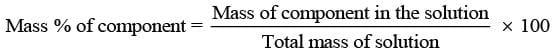Thus, the percentage by mass means the mass of the solute in grams present in 100g of the solution.
(ii) V/V (volume percentage) is defined as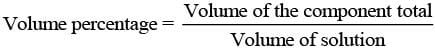Thus, volume percentage means the volume of the liquid solute in cm3 present in 100 cm3 of the solution.
(iii) w/V (mass by volume percentage) = mass of solute dissolved in 100 mL of solution.
(iv) ppm (parts per million): This parameter is used to express the concentration of very dilute solution.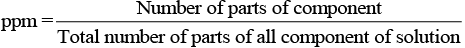(v) x (mole fraction): Mole fraction is a unitless quantity used to determine extent of any particular component present in total solution.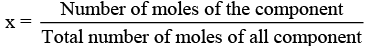(vi) M (molarity): Number of moles of solute dissolved in per litre of solution is known as molarity.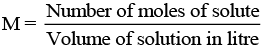(vii) m (Molality): Molality of any solution can be defined as number of moles of solute dissolved in per kg of solvent.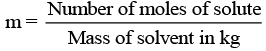Q.56. Using Raoult’s law explain how the total vapour pressure over the solution is related to mole fraction of components in the following solutions.
(i) CHCl3 (l) and CH2Cl2(l)
(ii) NaCl(s) and H2O (l)
Ans. According to Raoult's law for any solution the partial vapour pressure of each volatile component in the solution is directly proportional to its mole fraction.
p1 = P°1x1
(a) CHCl3 (l) and CH2Cl2 (l) both are volatile components.
Hence, for a binary solution in which both components are volatile liquids, the total pressure will be
p = p1 + p2 = x1p°1 + x22
= x11 + (1-x1)p°2
=(p°1 - p°2)x1 + p°2
Where, p = total vapour pressure
p1 = partial vapour pressure of component 1
p2 = partial vapour pressure of component 2
(b) NaCl (s) and H2O (l) both are non-volatile components. Hence, for a solution containing nonvolatile solute, the Raoult's law is applicable only to vaporisable component and total vapour pressure can be written as
p = p1 = x11
where p°1 reresents the vapour pressure of pure H2O(l).

Q.57. Explain the terms ideal and non-ideal solutions in the light of forces of interactions operating between molecules in liquid solutions.
Ans. The solutions which obey Raoult's law over the entire range of concentration are known as ideal solutions. In case of an ideal solution, enthalpy of mixing, ΔHmix = 0 and volume change on mixing, ΔVmix = 0. The ideal behaviour of the solutions can be explained by considering two components A and B.
In pure components, the intermolecular attractive interactions will be of A—A type and B—B type, whereas in the binary solutions in addition to these two, A—B type of interaction will also be present. If A—A and B—B intermolecular forces are nearly equal to those between A—B, this leads to the formation of ideal solution.
Example: solution of n-hexane and n-heptane.
When a solution does not obey-Raoult's law over the entire range of concentration, then it is called nonideal solution. The vapour pressure of such a solution is either higher or lower, than that predicted by Raoult's law.
If it is higher, the solution exhibits positive deviation and if it is lower it exhibits negative deviation from Raoult's law. In case of positive deviation, A—B interactions are weaker than those between A—A or B—B. i.e., the attractive forces between solute solvent molecules are weaker than those between solute-solute and solvent-solvent molecules.
Example: mixture of ethanol and acetone.
For such solutions ΔHmixing = + ve and ΔVmixing = + ve
On the other hand, in case of negative deviation the intermolecular attractive forces between A—A and B—B are weaker than those between A—B molecules. Thus, the escaping tendency of A and B types of molecules from the solution becomes less than from the pure liquids i.e., mixture of chloroform and acetone.
For such solutions ΔHmix = - ve and ΔVmix = - ve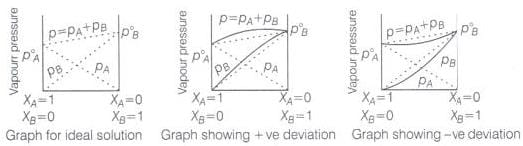Q.58. Why is it not possible to obtain pure ethanol by fractional distillation? What general name is given to binary mixtures which show deviation from Raoult’s law and whose components cannot be separated by fractional distillation. How many types of such mixtures are there?
Ans. The solution or mixture having same composition in liquid as well as in vapour phase and boils at a constant temperature is known as azeotropes. Due to constant composition it can't be separated by fractional distillation. There are two types of azeotropes
(a) Minimum boiling azeotropes: Solutions which show large positive deviation from Raoult's law form minimum boiling azeotropes at a specific composition.
Example: ethanol —water mixture
(b) Maximum boiling azeotropes: Solutions which show large negative deviation from Raoult's law form maximum boiling azeotropes.
Example: solution having composition 68% HNO3 and 32% water by mass.

Q.59. When kept in water, raisin swells in size. Name and explain the phenomenon involved with the help of a diagram. Give three applications of the phenomenon.

Ans. This phenomenon is called endo osmosis, i.e., movement of water inside the raisin and shown with the help of diagram as the process of osmosis is of immense biological as well as industrially important. It is evident from the following examples.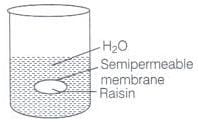(i) Movement of water from soil into plant roots and subsequently into upper portion of the plant is partly due to osmosis.
(ii) Preservation of meat against bacterial action by addition of salt.
(iii) Preservation of fruits against bacterial action by adding sugar. Bacterium in canned fruit loses water through the process of osmosis and become inactive.
(iv) Reverse-osmosis is used in desalination of water.

Q.60. Discuss biological and industrial importance of osmosis.
Ans.
(i) In animals, water moves into different parts of the body under the effect of the process of osmosis.
(ii) Stretching of leaves, flower, etc., is also controlled by osmosis.
(iii) Osmosis helps in rapid growth of the plants and germination of seeds.
(iv) Different movements of plants such as opening and closing of flowers etc, are controlled by osmosis.

Q.61. How can you remove the hard calcium carbonate layer of the egg without damaging its semipermeable membrane? Can this egg be inserted into a bottle with a narrow neck without distorting its shape? Explain the process involved.
Ans. When egg is placed in mineral acid solution outer shell of egg dissolves.
Egg is now removed and placed in hypertonic solution. Size of egg gets reduced and egg shrivels due to osmosis. Egg is now placed in a bottle with narrow neck. Finally on adding hypotonic solution egg regains its shape due to osmosis.
Diagrammatically it can be represented as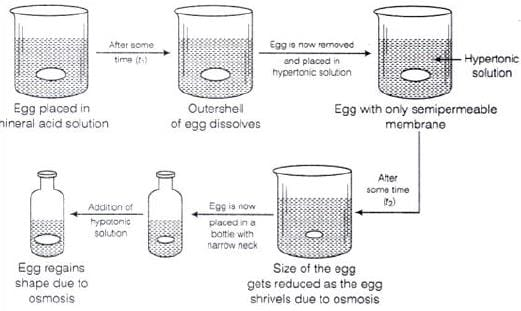Q.62. Why is the mass determined by measuring a colligative property in case of some solutes abnormal ? Discuss it with the help of Van’t Hoff factor.

Ans. Certain compounds when dissolved in suitable solvents either dissociate or associate.
Example: ethanoic acid dimerises in benzene due to H-bonding.
while in water, it dissociates and forms ions. As a result, the number of chemical species in solution increases or decreases as compared to the number of chemical species of solute added to form the solution.
Since, the magnitude of colligative property depends on the number of solute particles, it is expected that the molar mass determined on the basis of colligative properties will either higher or lower than the expected value or the normal value and is called abnormal molar mass.
In order to account for the extent of dissociation or association of molecules in solution, van't Hoff introduced a factor, i, known as the van't Hoff factor.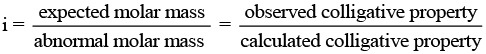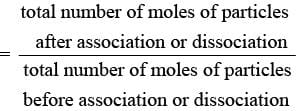The document NCERT Exemplar (Part - 2) - Solutions Notes | Study Additional Documents and Tests for JEE - JEE is a part of the JEE Course Additional Documents and Tests for JEE.
All you need of JEE at this link: JEE

## Additional Documents and Tests for JEE

11 videos|159 docs|14 tests
 Use Code STAYHOME200 and get INR 200 additional OFF

## Additional Documents and Tests for JEE

11 videos|159 docs|14 tests

Track your progress, build streaks, highlight & save important lessons and more!

,

,

,

,

,

,

,

,

,

,

,

,

,

,

,

,

,

,

,

,

,

;